# GOE and Graphs

To a graph one can associate a combinatorial Laplacian, which operates on functions on the vertices by giving the sum of the differences between the values of a function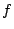at the vertex and its neighbors: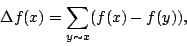the sum being over all neighbours of the vertex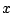. There are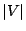eigenvalues. If we take a sequence of graphs such that the number of edges tends to infinity, then under certain conditions there is a limiting density of states analogous to Weyl's law. This gives a mean counting function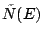, the expected number of levels below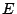. If, as usual, we unfold the sequence, then we get a sequence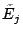with mean spacing unity. Put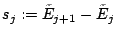. Then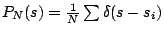is the distribution function of the spacings and is called the level spacing distribution of the graph.

A question that arises is to study the level spacing distribution of certain families of graphs. One such family is the family of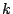-regular graphs. A graph is-regular if each vertex has exactlyneighbours. For this family numerical evidence  indicates that the resulting family of graphs have GOE spacings. Indeed in  the authors conjecture that for fixed degree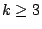, the eigenvalues of the generic-regular graph on a large number of vertices have fluctuations which tend to those of GOE.

On the other hand certain classes of graphs are known (for example 4-regular Cayley graphs on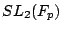,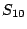, and large cyclic groups) where numerics suggest that the eigenvalue distribution is Poisson [ MR 2000g:05072]. Cayley graphs onare naturally thought of as discrete approximations to the spectral behaviour in the continuous setting of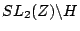, where computations indicate that the spacing distribution should be also Poisson. In all cases where the distribution seems to be Poisson, there are symmetries or degeneracies (eigenvalues occurring with a high multiplicity).

 D. Jakobson, S.D. Miller, I. Rivin and Z. Rudnick, Eigenvalue spacings for regular graphs, in Emerging applications of number theory.

Back to the main index for L-functions and Random Matrix Theory.